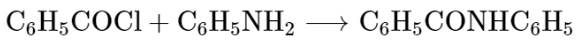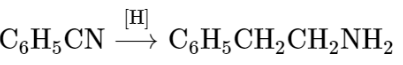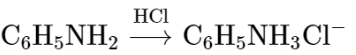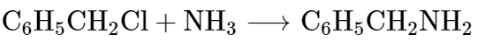# Which of the following reaction is an example of ammonolysis?Question:

Which of the following reaction is an example of ammonolysis?

1.2.3.4.Correct Option: , 4

Solution:

The process of cleavage of the $\mathrm{C}-\mathrm{X}$ bond by Ammonia molecule is known as ammonolysis.

$\mathrm{Ex}: \mathrm{R}-\mathrm{CH}_{2}-\mathrm{Cl}+\ddot{\mathrm{N} H}_{3} \longrightarrow \mathrm{R}-\mathrm{CH}_{2}-\mathrm{NH}_{2}$